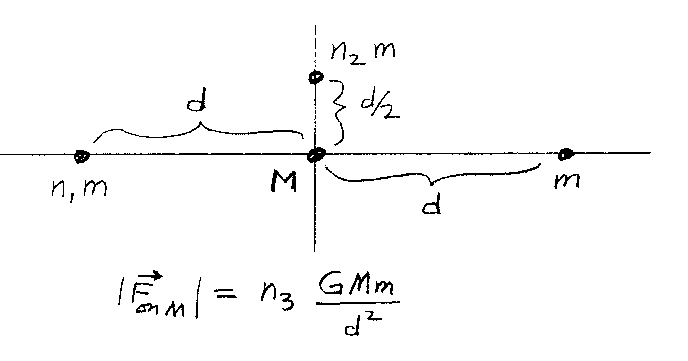Problem B3: There are 4 small objects located in the x-y plane. One object of mass m is at (d,0). Another object of mass (n1m) is located at (-d,0). A third object of mass (n2m) is located at (0,d/2) as shown in the figure below. What is the magnitude of the graviational force on the object of mass M placed at the origin? If the magnitude of the force on the object at the origin is F = n3 GMm/d2, what is n3? Note that all the integers are unitless.n1 = n2 = Input n3:

If you are currently in my class, you can record your grade by entering your name and student ID number (without the leading zeros) below and clicking on "record grade".
 First Name = Last Name = ID = Problem: